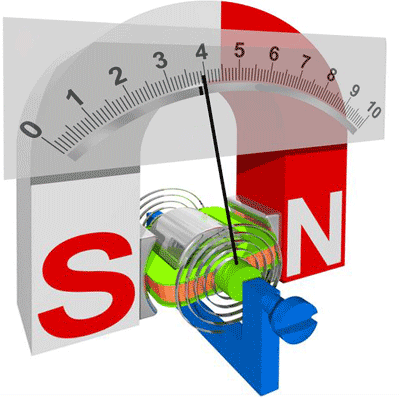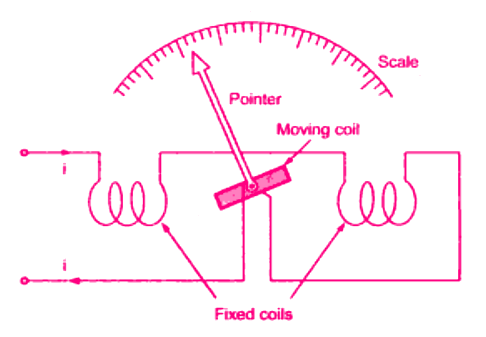# Ammeter Vs Voltmeter: What’s The DifferenceIf you are into electrical works, you must have heard of ammeter and voltmeter. Both of them are devices for measuring something. Many confuse the two and think both are the same thing.

Also, there are some who just barely understand the difference. But there are a lot of things to know about the differences between an ammeter and a voltmeter.

An ammeter is a device to use the amount of electrical current in a circuit. The voltmeter measures the voltage difference between two points in a circuit. Voltage is what determines the flow of current as we all know current flows from high voltage to low voltage area. There are tons of other differences between the two and their practical implementation also differs.

There are different types of ammeters and voltmeters. Their method of use also differs.

For someone in the work field of electricity, knowing the fundamentals of each as well as detailed differences between the two is very crucial. So that is my attempt today.

If you have any confusion between ammeter and voltmeter, you won’t be anymore after reading this article.

Contents

## What is Ammeter?

Electricity heats and lights our homes, start our cars, powers all of our electronic devices, etc. sometimes we may need to measure the amount of electricity flowing through the devices of our day-to-day use.

The ammeter can measure the electric current of a circuit. The name derives from ampere, the unit of measurement for electric current. You will often see that ampere is abbreviated as the amp.

Don’t worry, they are the same thing. A multimeter is a similar device. You can measure current and other things with a multimeter.

The ideal resistance of an ammeter should be zero so that the entire current of a circuit can flow through it. But practically, every ammeter has a small amount of resistance. The measuring capacity of an ammeter depends on the amount of resistance.

Capital A (A) is the symbolic representation of an ammeter in a circuit. Ammeter is connected to a circuit in series so that all the electricity of the circuit can pass through the ammeter to get a near accurate reading.

## Types of Ammeters

There are 4 types of ammeters. They are permanent moving coil ammeter, moving iron ammeter, electro-dynamometer ammeter, rectifier type ammeter.

Let’s have a brief look at them:

### Permanent Moving Coil AmmeterDirect current is measured using this ammeter.

In a permanent magnet moving coil (PMMC), the ammeter device is placed in the middle of the poles of a permanent magnet.

The coil’s deflection is dictated by the level of the current flow. PMCC ammeter is used for measuring direct current.

### Moving Iron AmmeterMoving iron ammeter is also known as moving coil ammeter. The coil on this one freely moves between two permanent magnet poles. When current flows through the coil, it begins to deflect at a specific angle.

The coil’s deflection is proportional to the current flowing through the ammeter. The moving iron ammeter is used for measuring both alternate and direct currents.

### Electro-dynamometer Ammeter

The electro-dynamometer is used to measure both alternating current and direct current. The accuracy of the electro-dynamometer is higher than PMMC and MI.### Rectifier Type Ammeter

The rectifier-type ammeter can measure alternating current. The instruments use a rectifying instrument to change the direction of the current for it to be sent to the PMMC instrument.

This instrument is used to measure the flow of current in a communication circuit.## How to Use an Ammeter

As voltmeter and ammeter are different, the ways to use them in a circuit are different as well. For example, to connect an ammeter in a circuit, you have to disconnect the circuit.

Following is a brief step by step guide on how to use an ammeter:

Step 1: Configure the ammeter’s current type and range.

Step 2: Check your ammeter’s internal fuse.

Step 3: Disconnect the circuit and connect the leads of the ammeter to the circuit.

Step 4: Reconnect the circuit and take the reading.

Step 5: Turn off the power and reconnect the circuit.

Step 6: find induction sensors, then position the sensor around the power supply wire.

Step 7: Set the ammeter to automatic.

Step 8: Take a reading and then remove the ammeter.

There’s a more detailed and illustrated guide on how to use an ammeter. You can find it here.

## What is Voltmeter?

You can measure the voltage of an electric circuit with a voltmeter. By using a voltmeter, you can detect the voltage or electric potential difference between two places.

Voltage measurement is essential for the development and maintenance of cutting-edge technology, but it also has more everyday and practical uses.

You might be confused about voltage. We all know that heat transfers from a high-temperature point to a low-temperature point. Gas flows from a high-pressure area to a low-pressure area. Similarly, electricity has to flow from somewhere to somewhere.

What determines direction? This is voltage. Electricity travels from high potential or high voltage area to low voltage area.

The voltmeter is connected in parallel to the circuit where you want to measure the voltage. The resistance of the voltmeter is greater than the ammeter so that current cannot flow through the meter and the exact amount of voltage can be measured.

One thing to remember is that you don’t need to disconnect the circuit to connect the voltmeter. Just knowing this information will save you from a lot of hassle.

## Types of Voltmeter

There are two main types of voltmeters depending on the output; analog voltmeters and digital voltmeters.

Based on construction, five types of voltmeters are rectifier type voltmeter, PMMC, MI voltmeter, electrodynamic voltmeter, induction type voltmeter.

Depending on the measurement, there are two types; ac voltmeter and dc voltmeter.

Analog Voltmeter: Analog voltmeter uses a pointer to give a reading. The display uses a need instead of a digital display.Digital Voltmeter: Unlike analog meters, digital voltmeters display both AC and DC voltage values. They give reading using digital numerical characters instead of needles or pointers.Rectifier Type Voltmeter: Rectifier type voltmeter measures alternating voltage and current.

PMMC Voltmeter: This type of voltmeter measures the flowing current by determining the deflection of the magnetic field.

MI voltmeter: MI voltmeters are of two types; attraction type and repulsion type.

Electro Dynamometer Type Voltmeter: When you place a current-carrying conductor inside a magnetic field, the conductor is subjected to a mechanical force due to the deflection in the conductor.

Induction Type of Voltmeter: Induction type voltmeter is the most common and widely used voltmeter.

## How to use a Voltmeter?

Using a voltmeter and using an ammeter is not the same. You can connect a voltmeter to a circuit without disconnecting it, unlike an ammeter: here is how to use a voltmeter in a circuit:

Step 1: Set the device to measure voltage

Step 2: Choose a range above the max anticipated voltage

Step 3: Insert the test leads

Step 4: Hold the probes with caution

Step 5: Touch the black test lead to one hole of the outlet.

Step 6: Touch the red test lead to another hole in the outlet.

Step 7: increase the range if you get an overload and adjust the voltmeter if need be

Here you can find an excellent guide on how to use a voltmeter. This guide has detailed descriptions and pictures to help you understand better.

## Difference Between Ammeter and Voltmeter

As you have already understood, ammeter and voltmeter are quite different. You can point out some differences yourself. A voltmeter measures voltage and an ammeter measure current.

The voltmeter is placed in parallel in a circuit whereas the ammeter is connected in series. The ideal resistance of the ammeter should be zero.

As far as the voltmeter is concerned, the ideal resistance is higher than the ammeter. Following are the summary of the key differences between the two:

Traits Voltmeter Ammeter
Measuring unit Voltmeter measures voltage and the unit is volt. Ammeter measures the total amount of current and the measuring unit is ampere.
Connection in a circuit A voltmeter is connected in parallel in a circuit. Ammeter is connected in series in a circuit.
Resistance The voltmeter has high resistance. Ammeter has low resistance.
Current amount A small amount of current passes through the voltmeter. Huge amount of current passes through the ammeter.
Circuit You don’t need to disconnect the circuit to attach the voltmeter. You have to disconnect the circuit to attach the ammeter.
Symbol The symbolic representation is V (capital V letter). The symbolic representation is A (capital A letter).

Related topics

does carbon fiber conduct electricity

does brass conduct electricity

does ice conduct electricity

does stainless steel conduct electricity

## Conclusion

Both ammeter and voltmeter have important usage in electrical works. Just knowing the basic differences is not enough. Their application and using methods are also quite different, so you have to know those as well to use these devices to their full potential.

A thorough knowledge about ammeter vs voltmeter is of utmost importance as an amateur or professional electrician. I believe I could point out the significant differences between the two and help you understand the application of each. Best of luck and hope you have a great day in the field!